Courses

# Multiple Correct MCQ Of Electromagnetic Induction And Alternating Current, Past Year Questions JEE Advance, Class 12, Physics

## 12 Questions MCQ Test Class 12 Physics 35 Years JEE Mains &Advance Past year Paper | Multiple Correct MCQ Of Electromagnetic Induction And Alternating Current, Past Year Questions JEE Advance, Class 12, Physics

Description
This mock test of Multiple Correct MCQ Of Electromagnetic Induction And Alternating Current, Past Year Questions JEE Advance, Class 12, Physics for JEE helps you for every JEE entrance exam. This contains 12 Multiple Choice Questions for JEE Multiple Correct MCQ Of Electromagnetic Induction And Alternating Current, Past Year Questions JEE Advance, Class 12, Physics (mcq) to study with solutions a complete question bank. The solved questions answers in this Multiple Correct MCQ Of Electromagnetic Induction And Alternating Current, Past Year Questions JEE Advance, Class 12, Physics quiz give you a good mix of easy questions and tough questions. JEE students definitely take this Multiple Correct MCQ Of Electromagnetic Induction And Alternating Current, Past Year Questions JEE Advance, Class 12, Physics exercise for a better result in the exam. You can find other Multiple Correct MCQ Of Electromagnetic Induction And Alternating Current, Past Year Questions JEE Advance, Class 12, Physics extra questions, long questions & short questions for JEE on EduRev as well by searching above.
*Multiple options can be correct
QUESTION: 1

### L, C and R represent the physical quantities inductance, capacitance and resistance respectively. Which of the following combinations have dimensions of frequency?

Solution:

KEY CONCEPT : The magnetic field due to a current flowing in a wire of finite length is given by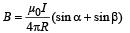Applying the above formula for AB for finding the field at O, we getacting perpendicular to the plane of paper upwards

∴ The total magnetic field due to current flowing through ABCD is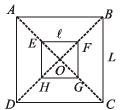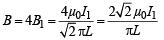The total flux passing through the square EFGH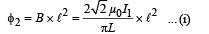[∵ ℓ > L and therefore, B can be assumed constant for ℓ2]

The flux through small square loop is directly proportional to the current passing through big square loop.

∴ φ2 ∝ I1 ⇒ φ = MI1 where M = Mutual Conductance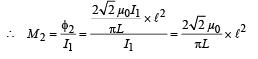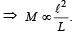*Multiple options can be correct
QUESTION: 2

### A conducting square loop of side L and resistance R moves in its plane with a uniform velocity v perpendicular to one of its sides. A magnetic induction B, constant in time and  space, pointing perpendicular and into the plane of the loop exists everywhere.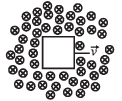The current induced in the loop is:

Solution:

NOTE : Since the rate of change of magnetic flux is zero, hence there will be  no net induced emf and hence no current flowing in the loop.

*Multiple options can be correct
QUESTION: 3

### Two different coils have self-inductances L1 = 8 mH and L2 = 2 mH. The current in one coil is increased at a constant rate. The current in the second coil is also increased at the same constant rate. At a certain instant of time, the power given to the two coils is the same. At that time, the current, the induced voltage and the energy stored in the first coil are i1, V1 and W1 respectively. Corresponding values for the second coil at the same instant are i2, V2 and W2 respectively. Then:

Solution: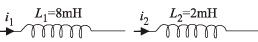Rate of change of current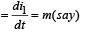Induced emf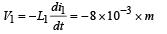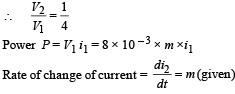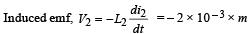Power  P = V2 i2 = 2 × 10 –3 × m × i2

Since Power is equal

∴ 8 × 10 – 3 × mi1 = 2 ×10 – 3 mi2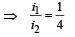...(i)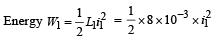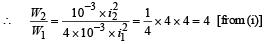*Multiple options can be correct
QUESTION: 4

A small square loop of wire of side l is placed inside a large square loop of wire of side L(L>>l). The loops are co-planar and their centres coincide. The mutual inductance of the system is proportional to

Solution:

KEY CONCEPT : The magnetic field due to a current flowing in a wire of finite length is given byApplying the above formula for AB for finding the field at O, we get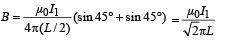acting perpendicular to the plane of paper upwards

∴  The total magnetic field due to current flowing through ABCD isThe total flux passing through the square EFGH[∵ ℓ  > L and therefore, B can be assumed constant for ℓ2]

The flux through small square loop is directly proportional to the current passing through big square loop.
∴ φ2 ∝ I1 ⇒ φ = MIwhere M = Mutual Conductance*Multiple options can be correct
QUESTION: 5

The SI unit of inductance, the henry, can be written as

Solution: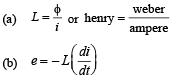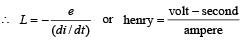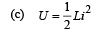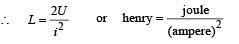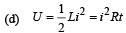∴ L = R.t or henry  = ohm-second.

*Multiple options can be correct
QUESTION: 6

A metal rod moves at a constant velocity in a direction perpendicular to its length. A constant, uniform magnetic field exists in space in a direction perpendicular to the rod as well as its velocity. Select the correct statement(s) from the following

Solution: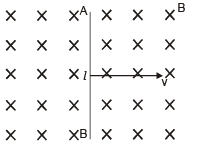A motional emf, e = Blv is induced in the rod. Or we can say a potential difference is induced between the two ends of the rod AB, with A at higher potential and B at lower potential.
Due to this potential difference, there is an electric field in the rod.

*Multiple options can be correct
QUESTION: 7

A series R– C circuit is connected to AC voltage source. Consider two cases; (A) when C is without a dielectric medium and (B) when C is filled with dielectric of constant 4.
The current IR through the resistor and voltage VC across the capacitor are compared in the two cases. Which of the following is/are true?

Solution: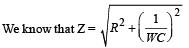The capacitance in case B is four times the capacitance in case A

∴ Impedance in case B is less then that of case A (ZB < ZA)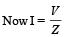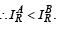option (a) is correct.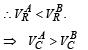[∵ If V is the applied potential difference access series R-C circuit then V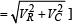∴ (c) is the correct option.

*Multiple options can be correct
QUESTION: 8

In the given circuit, the AC source has ω = 100 rad/s. Considering the inductor and capacitor to be ideal, the correct choice(s) is (are)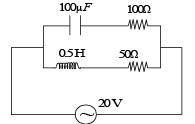Solution:

Impedance across AB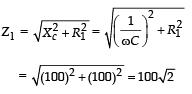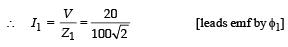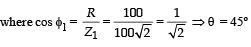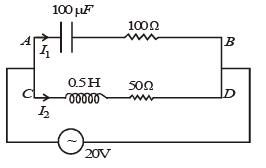Impedance across CD is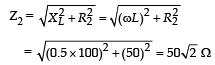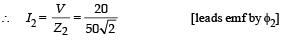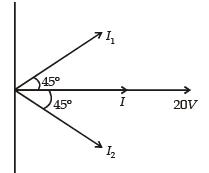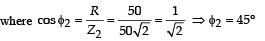∴The current I from the circuit is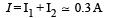*Multiple options can be correct
QUESTION: 9

A current carrying infinitely long wire is kept along the diameter of a circular wire loop, without touching it, the correct statement(s) is(are)

Solution:

Emf will be induced in the circular wire loop when flux through it changes with time.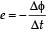when the current is constant, the flux changing through it will be zero.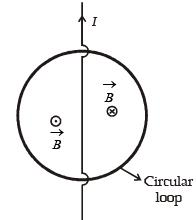When the current is decreasing at a steady rate then the change in the flux (decreasing inwards) on the right half of the wire is equal to the change in flux (decreasing outwards) on the left half of the wire such that Δφ through the circular loop is zero.

*Multiple options can be correct
QUESTION: 10

At time t = 0, terminal A in the circuit shown in the figure is connected to B by a key and an alternating current I(t) = I0cos (ωt), with I0 = 1 A and ω = 500 rad s–1 starts flowing in it with the initial direction shown in the figure.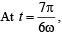the key is switched from B to D. Now onwards only A and D are connected. A total charge Q flows from the battery to charge the capacitor fully.
If C = 20 μF, R = 10 W and the battery is ideal with emf of 50 V, identify the correct statement(s).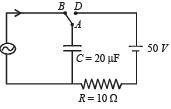Solution:

I = cos 500 t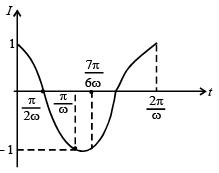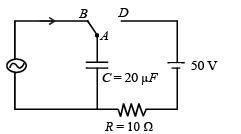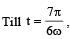the charge will be maximum at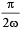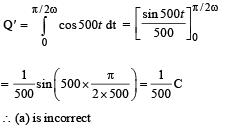From the graph it is clear that just before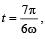the
current is in anticlockwise direction.

∴ (b) is incorrect

At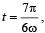the charge on the upper plate of capacitor
is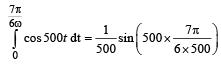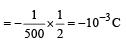Now applying KVL (when A is just connected to D)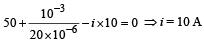∴ (c) is the correct option.
The maximum charge on C is Q = CV = 20 × 10–6 × 50 =10–3C
Therefore, the total charge flown = 2 × 10–3 C

∴ (d) is the correct option.

*Multiple options can be correct
QUESTION: 11

A conducting loop in the shape of a right angled isosceles triangle of height 10 cm is kept such that the 90° vertex is very close to an infinitely long conducting wire (see the figure). The wire is electrically insulated from the loop. The hypotenuse of the triangle is parallel to the wire. The current in the triangular loop is in counterclockwise direction and increased at a constant rate of 10 A s–1. Which of the following statement(s) is(are) true?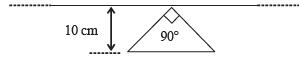Solution:

The flux passing through the triangular wire if i current flows through the inifinitely long conducting wire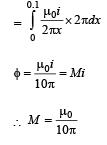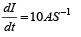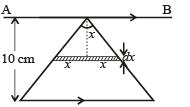Induced emf  in the wire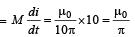As the current in the triangular wire is decreasing the induced current in AB is in the same direction as the  current in the hypotenuse of the triangular wire. Therefore force will be repulsive.

*Multiple options can be correct
QUESTION: 12

A rigid wire loop of square shape having side of length L and resistance R is moving along the x-axis with a constant velocity v0 in the plane of the paper. At t = 0, the right edge of the loop enters a region of length 3L where there is a uniform magnetic field B0 into the plane of the paper, as shown in the figure. For sufficiently large v0. the loop eventually crosses the region. Let x be the location of the right edge of the loop. Let v(x), I(x) and F(x) represent the velocity of the loop, current in the loop, and force on the loop, respectively, as a function of x. Counter-clockwise current is taken as positive.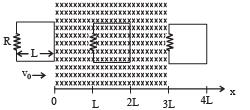Which of the following schematic plot(s) is (are) correct? (Ignore gravity)

Solution: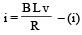[Counter clockwise directionwhile entering, Zero when completely inside and clockwise while exiting]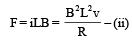[Toward left while entering and exiting and zero when completely inside]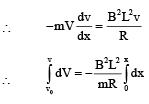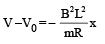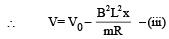[V decreases from x = 0 to x = L, remains constant for x = L to x= 3L again decreases from x=3L to x=4L] From (i) and (iii)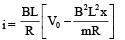[i decreases from x = 0 to x = L i becomes zero from x = L to x = 3L i changes direction and decreases from x = 3L to x = 4L]

These characteristics are shown in graph (a) and (b) only.